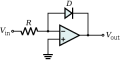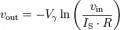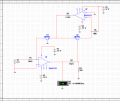# Problem with Logarithimic amplifier based on ua741

#### Electronicman

Joined Oct 18, 2011
11
I'm involved in an educational project and I want to design a logarithmic amplifier based on ua741. Following capture shows my circuit:Diode is 1N4148
Resistor Value is 33Kohms

And the results in lab and simulating don't match the results using the following formula:Vin= 6v
Vdd+=15v
Vdd-=-15v
Vt= 0.026 v
Is (Saturation Current = Reverse current) = 25 nA (I took from diode datasheet)
Even simulating results and lab results don't match.

Vin Simulating (Using multisim) ____Lab _____Formula
6v=> -0.552 _________________-0.523____ -0.231
5v=> -0.547 _________________ -0.516 ___ -0.226
4v=> -0.541 _________________-0.505_____-0.221

I don't know if I'm applying correctly the formula or if this is the formula I have to use.

Thank you very much in advance,

Joined Dec 26, 2010
2,148
The value you have for Is may or may not be appropriate. Some Spice models I have seen have values about an order of magnitude lower.

Note importantly, diodes are subject to an "ideality factor" N multiplying Vt, normally between 1 and 2.

The LTSpice model for 1N4148 gives Is=2.52nA and N=1.752.

Using these figures, you would have something like

Vout = -1.752*0.026V*ln(Vin/(2.52e-9A*33e3Ω))

Don't expect miracles, but it should be closer

#### Electronicman

Joined Oct 18, 2011
11
Using Is=2.52 nA and N=1.752 it gets closer but not exactly the same.
So Multisim uses these values?

How can it be more accurate? This circuit belongs to a Ac-Dc Converter based on True-RMS.

Joined Dec 26, 2010
2,148
Different samples of a general-purpose diode under the same conditions could easily differ by tens of mV, if not hundreds. A glance at the datasheet for some diodes will show that this is so.

There will also be a temperature coefficient of the forward voltage, typically of about -2mV/°C. The coefficient may change a little over a wide current range. To my mind rather counter-intuitively, this seems to grow bigger at smaller currents. Perhaps some device guru will come and explain why this is so - perhaps variation of the ideality factor?

Individual characterisation of the diode, and the use of a second diode at a known current would help to minimise these issues.

Preferably, the two diodes should be integrated onto the same silicon so that they their characteristics and junction temperatures will be as similar as possible.

I am not familiar with Multisim, but it may be interesting to wiew the diode model parameters, if these are accessible to the user.

Edit: See Page 2 of this datasheet. http://www.vishay.com/docs/81857/1n4148.pdf
Note that the maximum reverse current and the typical saturation current are not the same thing.

Last edited:

#### Electronicman

Joined Oct 18, 2011
11
The point is that I need to be more accurate. I need to know exactly what it's happening.

In the second page of the datasheet? I have only found the reverse current.

Diode:

MODEL 1N4148_2 d (
+ IS=6.89131e-09 RS=0.636257 N=1.82683 EG=1.15805
+ XTI=0.518861 BV=80 IBV=0.0001 CJO=9.99628e-13
+ VJ=0.942987 M=0.727538 FC=0.5 TT=4.33674e-09
+ KF=0 AF=1 )

I'm still not sure if I neeed to use this components to build a logarithmic amplifier. isn't it possible that it must be done using different electronic components?

Joined Dec 26, 2010
2,148
If you need to know exactly what is happening, you should look again at that datasheet. The graphs on it will show you much more than just the reverse current value. They will give you an appreciation of likely variabilities from device to device and over temperature. In addition, you will see that the typical reverse current behaviour is very far from the idealised constant Is of the diode model.

To help temperature effects and obtain a readily adjustable reference level, consider using two logarithmic amplifiers with a differential amplifier. One log circuit has a fixed input, the other handles the actual input. This will certainly help with temperature effects, and allows the reference level to be adjusted by altering the "fixed" current.

Using more closely matched diodes may be necessary - the use of elements from something like a transistor array might be better still.

Note also that the 741 op-amp has relatively poor input offset voltage and bias current performance, more modern devices could be much better.

Finally, setup gain and offset trims (using potentiometers, or preferably select-on test resistors) may be required for optimum accuracy.

In the end, you may require a level of accuracy which can only be obtained with an integrated logarithmic amplifier. If available to you, such a thing should offer better performance, but may be less educational.

#### ErnieM

Joined Apr 24, 2011
8,362
Isn't the diode backwards for a positive input?

Joined Dec 26, 2010
2,148
Isn't the diode backwards for a positive input?
With a positive input, the output goes negative, pulling current through the diode. I assume the OP is content to have a negative output. This is the configuration shown in a Wikipedia article, and it looks as if he may have copied it.

http://en.wikipedia.org/wiki/Log_amplifier

Edit: The diode can be replaced by a transistor. If a PNP device is used positive output can be obtained, but this requires negative input. My original statement that this worked with a positive input was wrong.
The Wikipedia article refers to this, calling it a trans diode, I have seen the configuration before, but don't remember it being called by that name.

The transistor dodge should desensitise the output to amplifier input offset voltage, as the base-emitter "diode" is referenced to common instead of the inverting input. I suppose though that wandering β with temperature and current must come into the mix a bit. Maybe not such an issue if β is large.

I was somewhat taken aback by the evident surprise expressed that it may be difficult to predict the performance of a randomly selected discrete diode to mV precision based on typical model data. If only...

Last edited:

#### Electronicman

Joined Oct 18, 2011
11
Thank you very much Adjuster and ErnieM.

You are right Adjuster, I took the configuration from Wikipedia. There is another configuration using a PNP transistor to obtain a positive output from a positive input.

Adjuster said: "Note that the maximum reverse current and the typical saturation current are not the same thing." So, which parameter do I have to take from the datasheet to use in my formula as Is? Is is not reprsented in any graphs.

Using an integrated logarithmic amplifier is the last option because it's an educational project and I would like to have all the steps. Before doing it I'll try another component than UA741 to get a better performance.

I didn't expect it to be so difficult. I mean, simulate such circuit with a simulation software and compare the results with the theorical formula.

#### DickCappels

Joined Aug 21, 2008
10,067
Yes, definitely, use an opamp with lower input bias current and offset voltage. Almost anything released in the last 25 years is better.

Diode saturation current is not on the specification sheets, and I don't know of a way to measure it directly, the parameter from a good SPICE model is probably the best you are going to find.

Reading through National Semiconductor Application Note AN-311 should help a lot.
http://www.national.com/an/AN/AN-311.pdf

And a couple of Bob Peas articles should help.
http://electronicdesign.com/article...s-all-this-logarithmic-stuff-anyhow-6068.aspx

Also by Bob Peas, which should be helpful:
http://www.national.com/rap/Story/vbe.html

Do not be overly concerned if you don't get exactly the same output as predicted by the formula; just feel lucky to get the right shape for now.

Joined Dec 26, 2010
2,148
I do not have much time for now, but must make a correction to something said earlier !

Please note that the transistor version as shown in Wikipedia remains inverting, so although it gives positive output, it requires negative input.

NB. What you need is specifically model data. Will try to say more later

#### Electronicman

Joined Oct 18, 2011
11
Because current saturation of diode and of transistor is very sensitive with temperature in the circuit above. I'll use the configuration explained in this article:

http://www-personal.engin.umd.umich...ICS II/01%c3-Misc Notes/Log&InverseLogAmp.pdf

Which is a variation of the one shown in the article mentioned by DickCappels:

http://www.national.com/an/AN/AN-30.pdf

I attach a screenshot of my circuit and simulation with multisim:Note that result in my simulation -1.188 don't match the result in the theorical formula using the same parameters.

Vout= - (K*T/Qe)(R2+R3/R3)ln{ (Vin/V+)(R6/R1) } = -0.42* ( ln{Vin} + 2,21) = -1,627 v (Vin=6 v)

Is it normal this variation in the results ??? I would be ok if my result was closer to the one of the formula.

Thank you very much in advance.

#### MrChips

Joined Oct 2, 2009
29,212
We had this thread before when someone was trying to do a multiply circuit with two logs and an anti-log circuit.
Log circuits are to be used in things such as compression circuits.
You cannot expect to get stable and accurate numerical results.

#### Electronicman

Joined Oct 18, 2011
11
I need the logarithmic amplifier because it's going to be a part of TRUE RMS-DC converter circuit which gives RMS value of any input signal.

If they are not stable, how are these converters supposed to be made? I think it must be a way to stabilize such circuits.

#### MrChips

Joined Oct 2, 2009
29,212
Aha. Now we know the truth.
As far as I know there are only two ways to determine true RMS value.
The first way is to determine the heating effect of the signal. This is done using a hot-wire ammeter.
The second way is to digitize the waveform and calculate the square root of the sum of the squares.

Joined Dec 26, 2010
2,148
Edit: more senile inexactitudes, to be ignored.

The power connections to your OPAs look wrong: both inputs to same polarity? I have more comments, but check this first.

Last edited:

Joined Dec 26, 2010
2,148
Aha. Now we know the truth.
As far as I know there are only two ways to determine true RMS value.
The first way is to determine the heating effect of the signal. This is done using a hot-wire ammeter.
The second way is to digitize the waveform and calculate the square root of the sum of the squares.
This is simply not correct. A logarithmic converter is an accepted way of generating a true-rms by first generating a square-law function.

Joined Dec 26, 2010
2,148
It may help to make some general points about diode equations, circuit simulation, and model parameter extraction.

Firstly, diode equations or simpler transistor models based on them cannot model all real devices exactly, and various simulators try to do better e.g. by adding more parameters to model such things as ohmic resistance. None of these models are perfect however, and different attempts at modelling the same device may give somewhat different results.

As an example, I enclose an LTSpice simulation result to a circuit similar to your original simple circuit. This gives 18.4mV variation of output for input changing from 4V to 6V, similar to the 18mV in your lab result, although the absolute voltages are not identical. Your Multisim result shows only 11mV difference: I cannot however say if this reflects a difference in the models, or some detail of how the simulations were set up. My version does not use the LM741, as I don't have a model, but I suspect this has negligible effect on the slope.

Some datasheet values such as leakage currents are unsuitable as model parameters. Is in particular needs to be separated from non-ideal surface leakage effects which may dominate overall leakage current values. The latter are in any case often worst-case values, not typical. Better accuracy might actually be obtained by using the forward conduction values as input to a curve-fitting exercise. Preferably, model parameter values are obtained directly from device manufacturers or suppliers of simulation software.

One thing that is certain is that beyond any inherent limitations of models, individual semiconductor devices vary, setting limits on the accuracy of prediction. This is, I think a valuable lesson. That said, simulation is not always a hopeless exercise. In case of really odd results, check your assumptions. A wrong coefficient or a schematic entry error is always a possibility.

Finally, note that device variability may be significant in circuits using devices to compensate each other for temperature, such as your improved log amplifier. As well as making sure that the devices are at the same temperature, it may be important to have the devices well matched. For this reason, the use of elements of an IC array may be preferred, if practicable.

#### Electronicman

Joined Oct 18, 2011
11
I think the power connections are ok. Both OAs have +/- 12 v. -12 V in pin 4 and 12V in pin 7, you mean that? What comments do you have more?